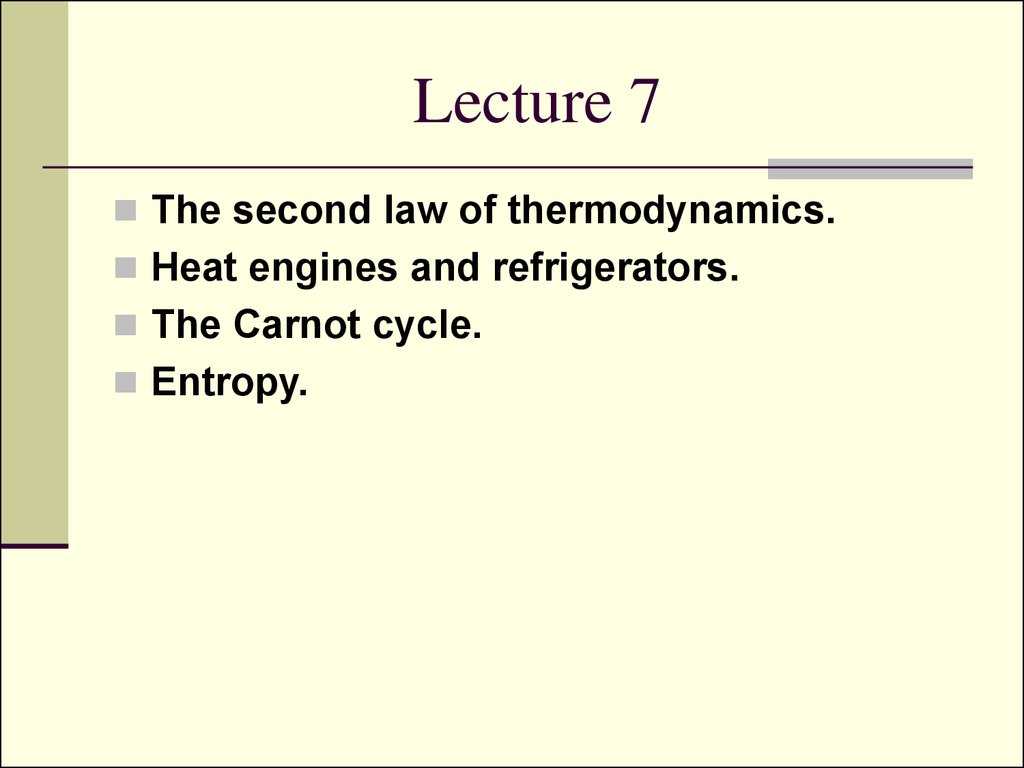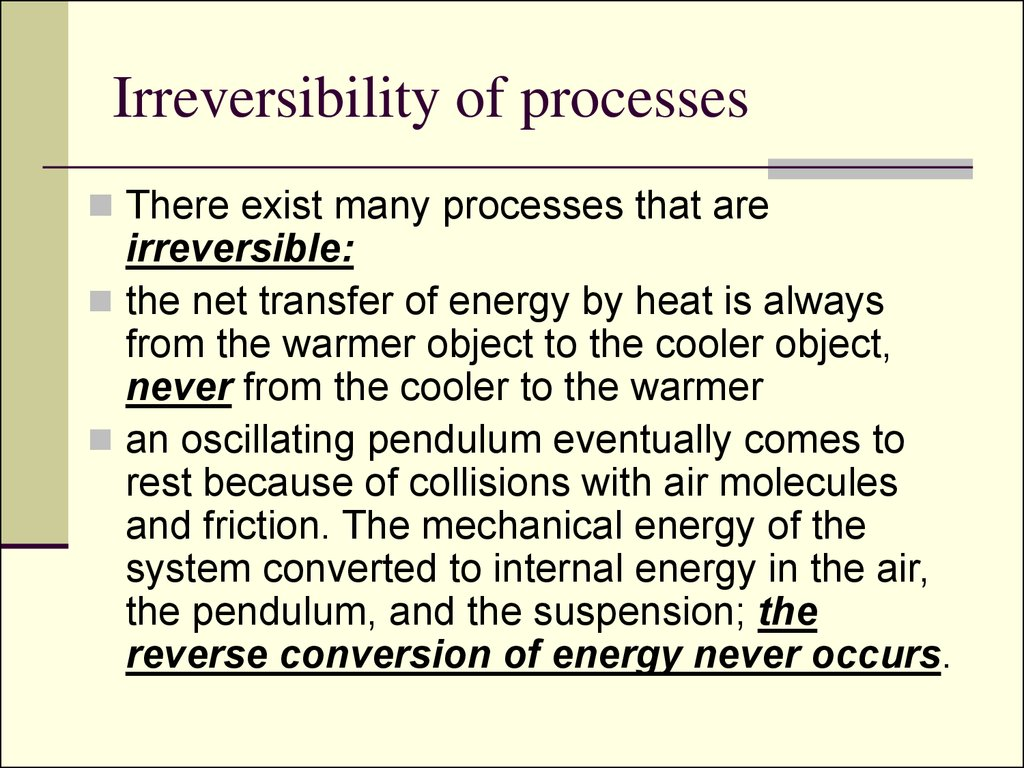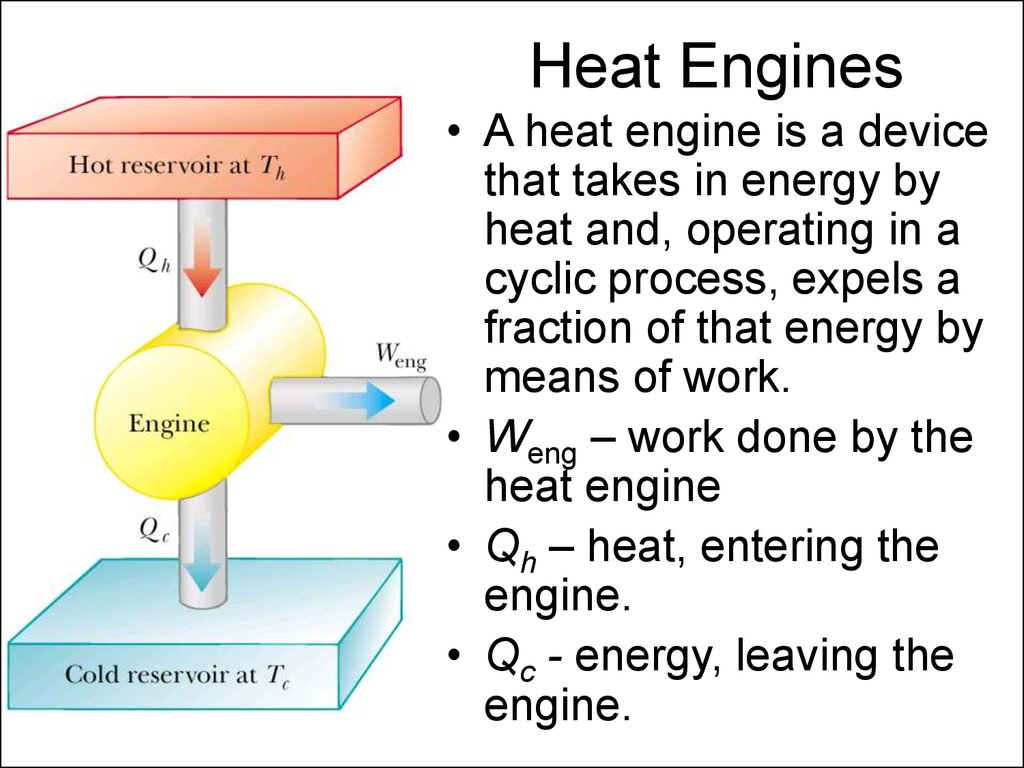# The second law of thermodynamics

## 1. Lecture 7

The second law of thermodynamics.
Heat engines and refrigerators.
The Carnot cycle.
Entropy.

## 2. Irreversibility of processes

There exist many processes that are
irreversible:
the net transfer of energy by heat is always
from the warmer object to the cooler object,
never from the cooler to the warmer
an oscillating pendulum eventually comes to
rest because of collisions with air molecules
and friction. The mechanical energy of the
system converted to internal energy in the air,
the pendulum, and the suspension; the
reverse conversion of energy never occurs.

## 3. Heat Engines

• A heat engine is a device
that takes in energy by
heat and, operating in a
cyclic process, expels a
fraction of that energy by
means of work.
• Weng – work done by the
heat engine
• Qh – heat, entering the
engine.
• Qc - energy, leaving the
engine.

## 4. Thermal Efficiency of a Heat Engine

Good Automobile engine efficiency is about 20%
Diesel engine efficiency is about 35%-40%

## 5. Heat Pumps or Refrigerators

In a heat engine a fraction of heat from the
hot reservoir is used to perform work.
In a refrigerator or a heat pump work is
used to take heat from the cold reservoir and
directed to the hot reservoir.

## 6. Refrigerator

• W – work done
on the heat pump
• Qh – heat, put
into the hot
reservoir.
• Qc - heat, taken
from the cold
reservoir.

## 7. Coefficient of performance of a refrigerator

The effectiveness of a refrigerator is
described in terms of a number called the
coefficient of performance (COP).
COP = Qc /(Qh - Qc) = Qc /W
Good refrigerate COP is about 5-6.

## 8. The Second Law of Thermodynamics

The Kelvin form:
It is impossible to construct a cyclic
engine that converts thermal energy
from a body into an equivalent amount
of mechanical work without a further
change in its surroundings.
Thus it says that for a heat engine it’s
impossible for QC=0, or heat engine
efficiency e=100%.

## 9. The Second Law of Thermodynamics

The Clausius form:
It is impossible to construct a cyclic
engine which only effect is to transfer
thermal energy from a colder body to a
hotter body.
Thus for refrigerator it’s impossible that W=0,
or COP = .

## 10. Carnot cycle

1. A-B isothermal
expansion
expansion
3. C-D isothermal
compression
compression

## 11. Carnot Efficiency

Using the equation of state and the first law of
thermodynamics we can easily find that (look
Servay p.678; Fishbane p.581):
Let’s prove it: During the isothermal
expansion (process A → B), the work done by
a gas during an isothermal process:

## 12.

• So, the work done on a gas during an
isothermal process A → B is:
(1)
• Similarly, for isothermal C → D:
(2)
Deviding (2) over (1):
(3)

## 13.

So, statement (3) gives us:

## 14.

So, using the last expression and the expression for efficiency:
Thus we have proved that the Carnot Efficiency equals
1.
2.
3.
Carnot Engine does not depend on the use of the ideal gas
as a working substance.
Carnot Engine is Reversible – it can be used as a
refrigerator or heat pump.
Carnot Cycle is the most efficient cycle for given two
temperatures Th and Tc.

## 15. Carnot theorem

The Carnot engine is the most
efficient engine possible that
operates between any two given
temperatures.
(look Servay p.675; Fishbane p.584)

## 16. Carnot Theorem Proof

Let’s prove it from the contrary:
let’s have Carnot engine A to be
more efficient than Carnot engine
B, they run together, engine B is
operating in reverse. We adjust A
to take Qh and B to give the same
Qh to the hot reservoir Th. Thus we get QcB –
QcA=DW. It means that we take energy from cold
reservoir to produce work DW. But it violates the
second law of thermodynamics.

## 17. Entropy

Measures the amount of disorder in thermal system.
It is a function of state, and only changes in entropy
have physical significance.
Entropy changes are path independent.
Another statement of the Second Law of
Thermodynamics: The total entropy of an isolated
system that undergoes a change cannot decrease.
For infinitesimal changes:

## 18. Entropy change calculations

Entropy is a state variable, the change in
entropy during a process depends only on the
end points and therefore is independent of
the actual path followed. Consequently the
entropy change for an irreversible process
can be determined by calculating the entropy
change for a reversible process that connects
the same initial and final states.

## 19.

• So for infinitesimal changes:
• The subscript r on the quantity dQr means that the transferred
energy is to be measured along a reversible path, even though
the system may actually have followed some irreversible path.
When energy is absorbed by the system, dQr is positive and
the entropy of the system increases. When energy is expelled
by the system, dQr is negative and the entropy of the system
decreases.
• Thus, it’s possible to choose a particular reversible path over
which to evaluate the entropy in place of the actual path, as
long as the initial and final states are the same for both paths.

## 20. Change of Entropy in a Carnot Cycle

Carnot engine operates between the temperatures Tc
and Th. In one cycle, the engine takes in energy Qh
from the hot reservoir and expels energy Qc to the
cold reservoir. These energy transfers occur only
during the isothermal portions of the Carnot cycle
thus the constant temperature can be brought out in
Thus, the total change in entropy for one cycle is

## 21. Reversibility of Carno Cycle

Using equality, proved for the Carnot Cycle (slide N13):
We eventually find that in Carno Cycle:
DS=0

## 22. Reversible Cycle

Now consider a system taken through an arbitrary (non-Carnot)
reversible cycle. Because entropy is a state variable —and
hence depends only on the properties of a given equilibrium
state —we conclude that
DS=0
for any reversible cycle. In general, we can write this condition
in the mathematical form
the symbol
path.
indicates that the integration is over a closed

## 23. Ideal Gas Reversible Process

Suppose that an ideal gas undergoes a quasi-static,
reversible process from an initial state Ti, Vi to a final
state Tf, Vf .
dQr = ΔU + W,
Work:
W=pdV,
Internal Energy change:
ΔU=nCvdT, (n – moles number)
Equation of state for an Ideal Gas: P=nRT/V,
Thus:
dQr = nCvdT + nRTdV/V
Then, dividing the last equation by T, and integrating we
get the next formula:
1st law of thermodynamics:

## 24.

- This expression demonstrates that DS
depends only on the initial and final states and
is independent of the path between the states.
The only claim is for the path to be reversible.
- DS can be positive or negative
- For a cyclic process (Ti= Tf, Vi = Vf), DS=0.
This is further evidence that entropy is a state
variable.

## 25. The Second Law of Thermodynamics

The total entropy of an isolated system
that undergoes a change cannot decrease.
If the process is irreversible, then the total
entropy of an isolated system always
increases. In a reversible process, the total
entropy of an isolated system remains
constant.

## 26. Microscopic States

Every macrostate can be realized by a number of
microstates.
Each molecule occupies some microscopic volume Vm.
The total number of possible locations of a single
molecule in a macroscopic volume V is the ratio
w =V/Vm.
Number w represents the number of ways that the
molecule can be placed in the volume, or the number of
microstates, which is equivalent to the number of
available locations.
If there are N molecules in volume V, then there are
W = wN = (V /Vm)N
microstates, corresponding to N molecules in volume V.

## 27. Entropy on a Microscopic Scale

Let’s have an ideal gas expanding from Vi to Vf.
Then the numbers of microscopic states are:
For initial state: Wi = wiN = (Vi /Vm)N .
For final state: Wf = wfN = (Vf /Vm)N .
Now let’s find their ratio:
So we canceled unknown Vm.

## 28.

After further transformations:
n – number of moles, R=kbNa.
Then we use the equation for isothermal expansion
(look Servay, p.688):
Using the expression from the previous slide
we get:

## 29. Entropy is a measure of Disorder

The more microstates there are that correspond to
a given macrostate, the greater is the entropy of
that macrostate.
Thus, this equation indicates mathematically that
entropy is a measur measure of disorder. Although
in our discussion we used the specific example of
the free expansion of an ideal gas, a more rigorous
development of the statistical interpretation of
entropy would lead us to the same conclusion.

## 30. Independent Study

Reynold’s number, Poiseuille flow, viscosity,
turbulence (Fishbane p.481, Lecture on
physics Summary by Umarov).
Entropy Change in a Free Expansion.
(Servay p.688).
Entropy Change in Calorimetric Processes
(Servay p.689)